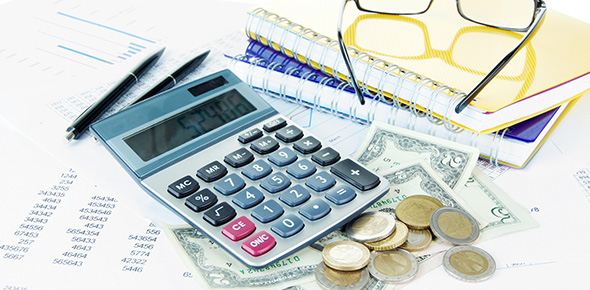# Accounting Quiz2

10 Questions | Total Attempts: 227SettingsRelated Topics
• 1.
Which accounts are remeasured (remeasurement method) using current exchange rates?
• A.

Cash, receivables, and most liabilities.

• B.

All assets and liabilities.

• C.

All noncurrent assets and liabilities.

• D.

All revenues and expenses.

• E.

All current assets and liabilities.

• 2.
Which accounts are translated (current method) using current exchange rates?
• A.

Cash, receivables, and most liabilities.

• B.

All assets and liabilities.

• C.

All noncurrent assets and liabilities.

• D.

All revenues and expenses.

• E.

All current assets and liabilities.

• 3.
Under the current rate method, inventory at market would be translated at what rate?
• A.

Current rate.

• B.

Average rate.

• C.

Composite amount.

• D.

Historical rate.

• E.

Beginning of the year rate.

• 4.
Under the current rate method, property, plant & equipment would be translated at what rate?
• A.

Current rate.

• B.

Average rate.

• C.

Composite amount.

• D.

Historical rate.

• E.

Beginning of the year rate.

• 5.
Under the current rate method, depreciation expense would be translated at what rate?
• A.

Current rate.

• B.

Average rate.

• C.

Composite amount.

• D.

Historical rate.

• E.

Beginning of the year rate.

• 6.
Under the current rate method, common stock would be translated at what rate?
• A.

Current rate.

• B.

Average rate.

• C.

Composite amount.

• D.

Historical rate.

• E.

Beginning of the year rate.

• 7.
Under the current rate method, retained earnings would be translated at what rate?
• A.

Current rate.

• B.

Average rate.

• C.

Composite amount.

• D.

Historical rate.

• E.

Beginning of the year rate.

• 8.
Under the current rate method, how would cost of goods sold be translated?
• A.

Current rate.

• B.

Average rate.

• C.

Composite amount.

• D.

Historical rate.

• E.

Beginning of the year rate.

• 9.
Under the remeasurement method, retained earnings would be remeasured at what rate?
• A.

Current rate.

• B.

Average rate.

• C.

Composite amount.

• D.

Historical rate.

• E.

Beginning of the year rate.

• 10.
Under the remeasurement method, depreciation expense would be remeasured at what rate?
• A.

Current rate.

• B.

Average rate.

• C.

Composite amount.

• D.

Historical rate.

• E.

Beginning of the year rate.